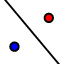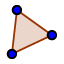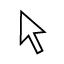Topic 3 Coordinate Plane Transformation Exploration Activity

Author:
Karen Rowe

Rule A: (x, y) -> (-x, y) Step 2

State the coordinates of the IMAGE triangle after applying the rule to the PREIMAGE triangle.

Rule A: (x, y) -> (-x, y) Step 3 Directions for Graphing IMAGE triangle on coordinate plane

Use the graph above to plot the image's coordinates. a. Use the INPUT line, located below the graph. to type in the IMAGE's coordinates for A. b. a point D should appear c. repeat for the B & C coordinates d. you should have three points (if the points are connected,) that look congruent to the pre-image triangle ABC. If it doesn't look congruent, check your points from Step 2.

Rule A: (x, y) -> (-x, y) Step 4 Identify the Transformation

Identify the transformation, which includes a line or its degree. - reflection over y = 0, also called the x-axis - reflection over the x = 0, also called the y-axis - rotate 90 degrees to the left, called a positive 90 degrees - rotate 90 degrees to the right, , called a positive 90 degrees - reflection over the y = 1x + 0, in simplified form y = x. - reflection over the y = -1x + 0, in simplified form y = -x. Copy and Paste your Answer

Rule A: (x, y) -> (-x, y) Step 5 Directions to Confirm your selected Transformation

Use Geogebra's transformation toolsto determine the transformation. Directions: For reflection over axis: select triangle then axis For reflection of y = x or y = -x, useto draw in y = x or y = -x. Then select the triangle then the line. For rotation, copy and paste either: rotate(polygon(A, B, C),90) or rotate(polygon(A, B, C),-90)

Rule A: (x, y) -> (-x, y) Step 6 Confirm your selected transformation is correct.

Use the Polygon toolto construct a scalene triangle ABC. The points will snap to integers. Use the select toolto select each individual vertex of the triangle. Move the mouse, which looks like a hand, to select. After pressing, select the Name & value. The coordinates for each vertex should appear.

Rule B: (x, y) -> (x, -y) Step 1 Graph

State the coordinates of the IMAGE triangle after applying the rule to the PREIMAGE triangle.

Rule B: (x, y) -> (x, -y) Step 2

Use the graph above to plot the image's coordinates. a. Use the INPUT line, located below the graph. to type in the IMAGE's coordinates for A. b. a point D should appear c. repeat for the B & C coordinates

Rule B: (x, y) -> (x, -y) Step 2: Directions for Image triangle

Select the transformation, which includes a line or its degree. - reflection over y = 0, also called the x-axis - reflection over the x = 0, also called the y-axis - rotate 90 degrees to the left, called a positive 90 degrees - rotate 90 degrees to the right, , called a positive 90 degrees - reflection over the y = 1x + 0, in simplified form y = x. - reflection over the y = -1x + 0, in simplified form y = -x. Copy and Paste your Answer

Rule B: (x, y) -> (x, -y) Step 3 Identify the Transformation for

Use Geogebra's transformation toolsto determine the transformation. Directions: For reflection over axis: select triangle then axis For reflection of y = x or y = -x, useto draw in y = x or y = -x. Then select the triangle then the line. For rotation, copy and paste either: rotate(polygon(A, B, C),90) or rotate(polygon(A, B, C),-90)

Rule C: (x, y)-> (-x, -y) Step 1

Use the graph above to plot the image's coordinates. a. Use the INPUT line, located below the graph. to type in the IMAGE's coordinates for A. b. a point D should appear c. repeat for the B & C coordinates

Rule C: (x, y)-> (-x, -y) Step 2 Directions for Graphing IMAGE triangle on coordinate plane

Select the transformation, which includes a line or its degree. - reflection over y = 0, also called the x-axis - reflection over the x = 0, also called the y-axis - rotate 90 degrees to the left, called a positive 90 degrees - rotate 90 degrees to the right, , called a positive 90 degrees - reflection over the y = 1x + 0, in simplified form y = x. - reflection over the y = -1x + 0, in simplified form y = -x. Copy and Paste your Answer

Rule C: (x, y)-> (-x, -y) Step 3 Identify the Transformation

Use Geogebra's transformation toolsto determine the transformation. Directions: For reflection over axis: select triangle then axis For reflection of y = x or y = -x, useto draw in y = x or y = -x. Then select the triangle then the line. For rotation, copy and paste either: rotate(polygon(A, B, C),90) or rotate(polygon(A, B, C),-90)

Rule D: (x, y) -> (y, x) Step 1

Use the graph above to plot the image's coordinates. a. Use the INPUT line, located below the graph. to type in the IMAGE's coordinates for A. b. a point D should appear c. repeat for the B & C coordinates

Rule D: (x, y) -> (y, x) Step 2 Directions for Graphing IMAGE triangle on coordinate plane

Use the graph above to plot the image's coordinates. a. Use the INPUT line, located below the graph. to type in the IMAGE's coordinates for A. b. a point D should appear c. repeat for the B & C coordinates

Rule D: (x, y) -> (y, x) Step 2 Directions for Graphing IMAGE triangle on coordinate plane

Use the graph above to plot the image's coordinates. a. Use the INPUT line, located below the graph. to type in the IMAGE's coordinates for A. b. a point D should appear c. repeat for the B & C coordinates

Rule D: (x, y) -> (y, x) Step 2 Directions for Graphing IMAGE triangle on coordinate plane

Select the transformation, which includes a line or its degree. - reflection over y = 0, also called the x-axis - reflection over the x = 0, also called the y-axis - rotate 90 degrees to the left, called a positive 90 degrees - rotate 90 degrees to the right, , called a positive 90 degrees - reflection over the y = 1x + 0, in simplified form y = x. - reflection over the y = -1x + 0, in simplified form y = -x. Copy and Paste your Answer

Rule D: (x, y) -> (y, x) Step 3 Identify the Transformation

Use Geogebra's transformation toolsto determine the transformation. Directions: For reflection over axis: select triangle then axis For reflection of y = x or y = -x, useto draw in y = x or y = -x. Then select the triangle then the line. For rotation, copy and paste either: rotate(polygon(A, B, C),90) or rotate(polygon(A, B, C),-90)

Rule D: (x, y) -> (y, x) Step 4 Directions to Confirm your selected Transformation

Select the transformation, which includes a line or its degree. - reflection over y = 0, also called the x-axis - reflection over the x = 0, also called the y-axis - rotate 90 degrees to the left, called a positive 90 degrees - rotate 90 degrees to the right, , called a positive 90 degrees - reflection over the y = 1x + 0, in simplified form y = x. - reflection over the y = -1x + 0, in simplified form y = -x. Copy and Paste your Answer

Rule E: (x, y)-> (-y, -x) Step 1

Select the transformation, which includes a line or its degree. - reflection over y = 0, also called the x-axis - reflection over the x = 0, also called the y-axis - rotate 90 degrees to the left, called a positive 90 degrees - rotate 90 degrees to the right, , called a positive 90 degrees - reflection over the y = 1x + 0, in simplified form y = x. - reflection over the y = -1x + 0, in simplified form y = -x. Copy and Paste your Answer

Rule E: (x, y)-> (-y, -x) Step 2 Directions for Graphing IMAGE triangle on coordinate plane

Use Geogebra's transformation toolsto determine the transformation. Directions: For reflection over axis: select triangle then axis For reflection of y = x or y = -x, useto draw in y = x or y = -x. Then select the triangle then the line. For rotation, copy and paste either: rotate(polygon(A, B, C),90) or rotate(polygon(A, B, C),-90)

Rule E: (x, y)-> (-y, -x) Step 3 Identify the Transformation

Use Geogebra's transformation toolsto determine the transformation. Directions: For reflection over axis: select triangle then axis For reflection of y = x or y = -x, useto draw in y = x or y = -x. Then select the triangle then the line. For rotation, copy and paste either: rotate(polygon(A, B, C),90) or rotate(polygon(A, B, C),-90)

Rule E: (x, y)-> (-y, -x) Step 4 Directions to Confirm your selected Transformation

Use Geogebra's transformation toolsto determine the transformation. Directions: For reflection over axis: select triangle then axis For reflection of y = x or y = -x, useto draw in y = x or y = -x. Then select the triangle then the line. For rotation, copy and paste either: rotate(polygon(A, B, C),90) or rotate(polygon(A, B, C),-90)

Rule E: (x, y)-> (-y, -x) Step 4 Directions to Confirm your selected Transformation

Use Geogebra's transformation toolsto determine the transformation. Directions: For reflection over axis: select triangle then axis For reflection of y = x or y = -x, useto draw in y = x or y = -x. Then select the triangle then the line. For rotation, copy and paste either: rotate(polygon(A, B, C),90) or rotate(polygon(A, B, C),-90)

Rule F: (x, y) -> ( y, -x) Step 1

Use the Polygon tool to construct a scalene triangle ABC. Place the points on whole coordinates. Then use the Polygon tool again with the coordinates after applying Rule G: (x, y) -> (-y, x) Use Geogebra's construction tools to determine which transformation and line or degree that corresponds to Rule G: (x, y) -> (-y, x)

Rule G: (x, y) -> (-y, x)

Use the Polygon tool to construct a scalene triangle ABC. Place the points on whole coordinates. Then use the Polygon tool again with the coordinates after applying Rule G: (x, y) -> (-y, x) Use Geogebra's construction tools to determine which transformation and line or degree that corresponds to Rule G: (x, y) -> (-y, x)

Rule F: (x, y) -> ( y, -x) Step 2 Directions for Graphing IMAGE triangle on coordinate plane

Use the graph above to plot the image's coordinates. a. Use the INPUT line, located below the graph. to type in the IMAGE's coordinates for A. b. a point D should appear c. repeat for the B & C coordinates

Rule C: (x, y)-> (-x, -y) Step 4 Directions to Confirm your selected Transformation

Select the transformation, which includes a line or its degree. - reflection over y = 0, also called the x-axis - reflection over the x = 0, also called the y-axis - rotate 90 degrees to the left, called a positive 90 degrees - rotate 90 degrees to the right, , called a positive 90 degrees - reflection over the y = 1x + 0, in simplified form y = x. - reflection over the y = -1x + 0, in simplified form y = -x. Copy and Paste your Answer

Rule F: (x, y) -> ( y, -x) Step 3 Identify the Transformation

Select the transformation, which includes a line or its degree. - reflection over y = 0, also called the x-axis - reflection over the x = 0, also called the y-axis - rotate 90 degrees to the left, called a positive 90 degrees - rotate 90 degrees to the right, , called a positive 90 degrees - reflection over the y = 1x + 0, in simplified form y = x. - reflection over the y = -1x + 0, in simplified form y = -x. Copy and Paste your Answer